• No products in the cart.

# A Brief Introduction to PySpark by Ben Weber

(23 views)

PySpark is a great language for performing exploratory data analysis at scale, building machine learning pipelines, and creating ETLs for a data platform. If you’re already familiar with Python and libraries such as Pandas, then PySpark is a great language to learn in order to create more scalable analyses and pipelines. The goal of this post is to show how to get up and running with PySpark and to perform common tasks.

We’ll use Databricks for a Spark environment, and the NHL dataset from Kaggle as a data source for analysis. This post shows how to read and write data into Spark dataframes, create transformations and aggregations of these frames, visualize results, and perform linear regression. I’ll also show how to mix regular Python code with PySpark in a scalable way, using Pandas UDFs. To keep things simple, we’ll focus on batch processing and avoid some of the complications that arise with streaming data pipelines.

The full notebook for this post is available on github.

### Environment

There’s a number of different options for getting up and running with Spark:

• Self Hosted: You can set up a cluster yourself using bare metal machines or virtual machines. Apache Ambari is a useful project for this option, but it’s not my recommended approach for getting up and running quickly.
• Cloud Providers: Most cloud providers offer Spark clusters: AWS has EMR and GCP has DataProc. I’ve blogged about DataProc in the past, and you can get to an interactive environment quicker than self-hosting.
• Vendor Solutions: Companies including Databricks and Cloudera provide Spark solutions, making it easy to get up and running with Spark.

The solution to use varies based on security, cost, and existing infrastructure. If you’re trying to get up and running with an environment to learn, then I would suggest using the Databricks Community Edition.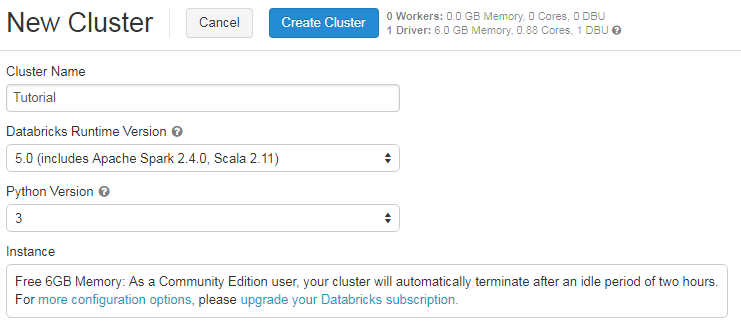With this environment, it’s easy to get up and running with a Spark cluster and notebook environment. For this tutorial, I created a cluster with the Spark 2.4 runtime and Python 3. To run the code in this post, you’ll need at least Spark version 2.3 for the Pandas UDFs functionality.

### Spark Dataframes

The key data type used in PySpark is the Spark dataframe. This object can be thought of as a table distributed across a cluster and has functionality that is similar to dataframes in R and Pandas. If you want to do distributed computation using PySpark, then you’ll need to perform operations on Spark dataframes, and not other python data types.

It is also possible to use Pandas dataframes when using Spark, by calling toPandas() on a Spark dataframe, which returns a pandas object. However, this function should generally be avoided except when working with small dataframes, because it pulls the entire object into memory on a single node.

One of the key differences between Pandas and Spark dataframes is eager versus lazy execution. In PySpark, operations are delayed until a result is actually needed in the pipeline. For example, you can specify operations for loading a data set from S3 and applying a number of transformations to the dataframe, but these operations won’t immediately be applied. Instead, a graph of transformations is recorded, and once the data is actually needed, for example when writing the results back to S3, then the transformations are applied as a single pipeline operation. This approach is used to avoid pulling the full data frame into memory and enables more effective processing across a cluster of machines. With Pandas dataframes, everything is pulled into memory, and every Pandas operation is immediately applied.

In general, it’s a best practice to avoid eager operations in Spark if possible, since it limits how much of your pipeline can be effectively distributed.

One of the first steps to learn when working with Spark is loading a data set into a dataframe. Once data has been loaded into a dataframe, you can apply transformations, perform analysis and modeling, create visualizations, and persist the results. In Python, you can load files directly from the local file system using Pandas:

```import pandas as pd

In PySpark, loading a CSV file is a little more complicated. In a distributed environment, there is no local storage and therefore a distributed file system such as HDFS, Databricks file store (DBFS), or S3 needs to be used to specify the path of the file.

Generally, when using PySpark I work with data in S3. Many databases provide an unload to S3 function, and it’s also possible to use the AWS console to move files from your local machine to S3. For this post, I’ll use the Databricks file system (DBFS), which provides paths in the form of /FileStore. The first step is to upload the CSV file you’d like to process.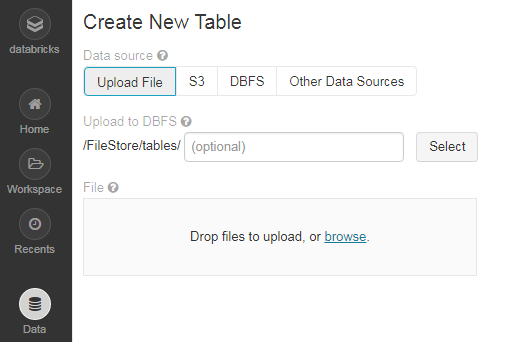The next step is to read the CSV file into a Spark dataframe as shown below. This code snippet specifies the path of the CSV file, and passes a number of arguments to the read function to process the file. The last step displays a subset of the loaded dataframe, similar to `df.head()` in Pandas.

```file_location = "/FileStore/tables/game_skater_stats.csv"
display(df)```

I prefer using the parquet format when working with Spark, because it is a file format that includes metadata about the column data types, offers file compression, and is a file format that is designed to work well with Spark. AVRO is another format that works well with Spark. The snippet below shows how to take the dataframe from the past snippet and save it as a parquet file on DBFS, and then reload the dataframe from the saved parquet file.

```df.write.save('/FileStore/parquet/game_skater_stats',
format='parquet')
display(df)```

The result of this step is the same, but the execution flow is significantly different. When reading CSV files into dataframes, Spark performs the operation in an eager mode, meaning that all of the data is loaded into memory before the next step begins execution, while a lazy approach is used when reading files in the parquet format. Generally, you want to avoid eager operations when working with Spark, and if I need to process large CSV files I’ll first transform the data set to parquet format before executing the rest of the pipeline.

Often you’ll need to process a large number of files, such as hundreds of parquet files located at a certain path or directory in DBFS. With Spark, you can include a wildcard in a path to process a collection of files. For example, you can load a batch of parquet files from S3 as follows:

`df = spark.read .load("s3a://my_bucket/game_skater_stats/*.parquet")`

This approach is useful if you have a seperate parquet file per day, or if there is a prior step in your pipeline that outputs hundreds of parquet files.

If you want to read data from a DataBase, such as Redshift, it’s a best practice to first unload the data to S3 before processing it with Spark. In Redshift, the unload command can be used to export data to S3 for processing:

```unload ('select * from data_to_process')
to 's3://my_bucket/game_data'
iam_role 'arn:aws:iam::123:role/RedshiftExport';```

There’s also libraries for databases, such as the spark-redshift, that make this process easier to perform.

### Writing Data

Similar to reading data with Spark, it’s not recommended to write data to local storage when using PySpark. Instead, you should used a distributed file system such as S3 or HDFS. If you going to be processing the results with Spark, then parquet is a good format to use for saving data frames. The snippet below shows how to save a dataframe to DBFS and S3 as parquet.

```# DBFS (Parquet)
df.write.save('/FileStore/parquet/game_stats',format='parquet')
# S3 (Parquet)
df.write.parquet("s3a://my_bucket/game_stats", mode="overwrite")```

When saving a dataframe in parquet format, it is often partitioned into multiple files, as shown in the image below.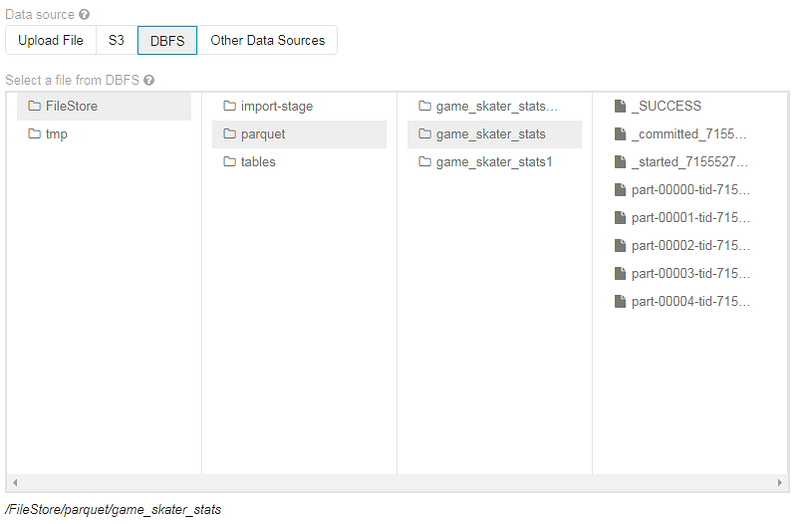If you need the results in a CSV file, then a slightly different output step is required. One of the main differences in this approach is that all of the data will be pulled to a single node before being output to CSV. This approach is recommended when you need to save a small dataframe and process it in a system outside of Spark. The snippet below shows how to save a dataframe as a single CSV file on DBFS and S3.

```# DBFS (CSV)
df.write.save('/FileStore/parquet/game_stats.csv', format='csv')
# S3 (CSV)
df.coalesce(1).write.format("com.databricks.spark.csv")

Another common output for Spark scripts is a NoSQL database such as Cassandra, DynamoDB, or Couchbase. This is outside the scope of this post, but one approach I’ve seen used in the past is writing a dataframe to S3, and then kicking off a loading process that tells the NoSQL system to load the data from the specified path on S3.

I’ve also omitted writing to a streaming output source, such as Kafka or Kinesis. These systems are more useful to use when using Spark Streaming.

### Transforming Data

Many different types of operations can be performed on Spark dataframes, much like the wide variety of operations that can be applied on Pandas dataframes. One of the ways of performing operations on Spark dataframes is via Spark SQL, which enables dataframes to be queried as if they were tables. The snippet below shows how to find top scoring players in the data set.

```df.createOrReplaceTempView("stats")
display(spark.sql("""
select player_id, sum(1) as games, sum(goals) as goals
from stats
group by 1
order by 3 desc
limit 5
"""))```

The result is a list of player IDs, number of game appearances, and total goals scored in these games. If we want to show the names of the players then we’d need to load an additional file, make it available as a temporary view, and then join it using Spark SQL.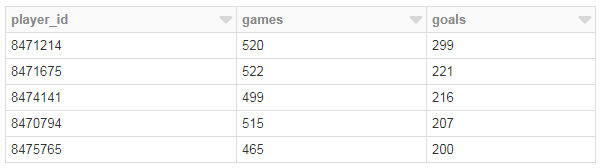In the snippet above, I’ve used the display command to output a sample of the data set, but it’s also possible to assign the results to another dataframe, which can be used in later steps in the pipeline. The code below shows how to perform these steps, where the first query results are assigned to a new dataframe which is then assigned to a temporary view and joined with a collection of player names.

```top_players = spark.sql("""
select player_id, sum(1) as games, sum(goals) as goals
from stats
group by 1
order by 3 desc
limit 5
""")```
```top_players.createOrReplaceTempView("top_players")
names.createOrReplaceTempView("names")```
```display(spark.sql("""
select p.player_id, goals, _c1 as First, _c2 as Last
from top_players p
join names n
on p.player_id = n._c0
order by 2 desc
"""))```

The result of this process is shown below, identifying Alex Ovechkin as a top scoring player in the NHL, based on the Kaggle data set.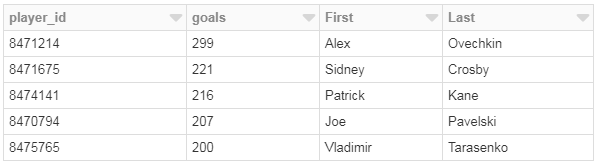There are Spark dataframe operations for common tasks such as adding new columns, dropping columns, performing joins, and calculating aggregate and analytics statistics, but when getting started it may be easier to perform these operations using Spark SQL. Also, it’s easier to port code from Python to PySpark if you’re already using libraries such as PandaSQL or framequery to manipulate Pandas dataframes using SQL.

Like most operations on Spark dataframes, Spark SQL operations are performed in a lazy execution mode, meaning that the SQL steps won’t be evaluated until a result is needed. Spark SQL provides a great way of digging into PySpark, without first needing to learn a new library for dataframes.

If you’re using Databricks, you can also create visualizations directly in a notebook, without explicitly using visualization libraries. For example, we can plot the average number of goals per game, using the Spark SQL code below.

```display(spark.sql("""
select cast(substring(game_id, 1, 4) || '-'
|| substring(game_id, 5, 2) || '-01' as Date) as month
, sum(goals)/count(distinct game_id) as goals_per_goal
from stats
group by 1
order by 1
"""))```

The initial output displayed in the Databricks notebook is a table of results, but we can use the plot functionality to transform the output into different visualizations, such as the bar chart shown below. This approach doesn’t support every visualization that a data scientist may need, but it does make it much easier to perform exploratory data analysis in Spark. If needed, we can use the toPandas() function to create a Pandas dataframe on the driver node, which means that any Python plotting library can be used for visualizing the results. However, this approach should be used for only small dataframes, since all of the data is eagerly fetched into memory on the driver node.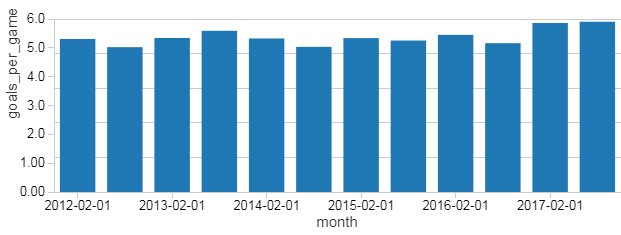I also looked at average goals per shot, for players with at least 5 goals.

```display(spark.sql("""
select cast(goals/shots * 50 as int)/50.0 as Goals_per_shot
,sum(1) as Players
from (
select player_id, sum(shots) as shots, sum(goals) as goals
from stats
group by 1
having goals >= 5
)
group by 1
order by 1
"""))```

The results for this transformation are shown in the chart below. Most of the players with at least 5 goals complete shots about 4% to 12% of the time.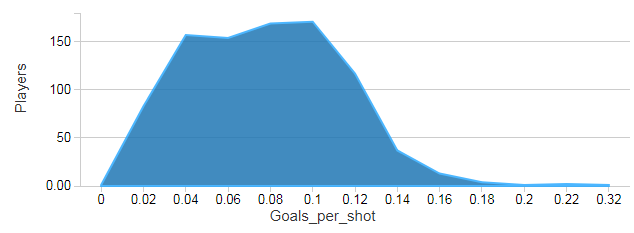### MLlib

One of the common use cases of Python for data scientists is building predictive models. While scikit-learn is great when working with pandas, it doesn’t scale to large data sets in a distributed environment (although there are ways for it to be parallelized with Spark). When building predictive models with PySpark and massive data sets, MLlib is the preferred library because it natively operates on Spark dataframes. Not every algorithm in scikit-learn is available in MLlib, but there is a wide variety of options covering many use cases.

In order to use one of the supervised algorithms in MLib, you need to set up your dataframe with a vector of features and a label as a scalar. Once prepared, you can use the fit function to train the model. The snippet below shows how to combine several of the columns in the dataframe into a single features vector using a VectorAssembler. We use the resulting dataframe to call the fit function and then generate summary statistics for the model.

```# MLlib imports
from pyspark.ml.feature import VectorAssembler
from pyspark.ml.regression import LinearRegression```
```# Create a vector representation for features
assembler = VectorAssembler(inputCols=['shots', 'hits', 'assists',
'penaltyMinutes','timeOnIce','takeaways'],outputCol="features")
train_df = assembler.transform(df)```
```# Fit a linear regression model
lr = LinearRegression(featuresCol = 'features', labelCol='goals')
lr_model = lr.fit(train_df)```
```# Output statistics
trainingSummary = lr_model.summary
print("Coefficients: " + str(lr_model.coefficients))
print("RMSE: %f" % trainingSummary.rootMeanSquaredError)
print("R2: %f" % trainingSummary.r2)```

The model predicts how many goals a player will score based on the number of shots, time in game, and other factors. However, the performance of this model is poor, it results in a root mean-squared error (RMSE) of 0.375 and an R-squared value of 0.125. The coefficient with the largest value was the shotscolumn, but this did not provide enough signal for the model to be accurate.

There’s a number of additional steps to consider when build an ML pipeline with PySpark, including training and testing data sets, hyperparameter tuning, and model storage. The snippet above is simply a starting point for getting started with MLlib.

### Pandas UDFs

One of the features in Spark that I’ve been using more recently is Pandas user-defined functions (UDFs), which enable you to perform distributed computing with Pandas dataframes within a Spark environment. The general way that these UDFs work is that you first partition a Spark dataframe using a groupby statement, and each partition is sent to a worker node and translated into a Pandas dataframe that gets passed to the UDF. The UDF then returns a transformed Pandas dataframe which is combined with all of the other partitions and then translated back to a Spark dataframe. The end result is really useful, you can use Python libraries that require Pandas but can now scale to massive data sets, as long as you have a good way of partitioning your dataframe. Pandas UDFs were introduced in Spark 2.3, and I’ll be talking about how we use this functionality at Zynga during Spark Summit 2019.

Curve fitting is a common task that I perform as a data scientist. The code snippet below shows how to perform curve fitting to describe the relationship between the number of shots and hits that a player records during the course of a game. The snippet shows how we can perform this task for a single player by calling toPandas() on a data set filtered to a single player. The output of this step is two parameters (linear regression coefficients) that attempt to describe the relationship between these variables.

```# Sample data for a player
sample_pd = spark.sql("""
select * from stats
where player_id = 8471214
""").toPandas()```
```# Import python libraries
from scipy.optimize import leastsq
import numpy as np```
```# Define a function to fit
def fit(params, x, y):
return (y - (params + x * params ))```
```# Fit the curve and show the results
result = leastsq(fit, [1, 0],
args=(sample_pd.shots, sample_pd.hits))
print(result)```

If we want to calculate this curve for every player and have a massive data set, then the toPandas() call will fail due to an out of memory exception. We can scale this operation to the entire data set by calling groupby() on the player_id, and then applying the Pandas UDF shown below. The function takes as input a Pandas dataframe that describes the gameplay statistics of a single player, and returns a summary dataframe that includes the player_idand fitted coefficients. Each of the summary Pandas dataframes are then combined into a Spark dataframe that is displayed at the end of the code snippet. One additional piece of setup for using Pandas UDFs is defining the schema for the resulting dataframe, where the schema describes the format of the Spark dataframe generated from the apply step.

```# Load necessary libraries
from pyspark.sql.functions import pandas_udf, PandasUDFType
from pyspark.sql.types import *
import pandas as pd```
```# Create the schema for the resulting data frame
schema = StructType([StructField('ID', LongType(), True),
StructField('p0', DoubleType(), True),
StructField('p1', DoubleType(), True)])```
```# Define the UDF, input and outputs are Pandas DFs
@pandas_udf(schema, PandasUDFType.GROUPED_MAP)
def analyze_player(sample_pd):```
```    # return empty params in not enough data
if (len(sample_pd.shots) <= 1):
return pd.DataFrame({'ID': [sample_pd.player_id],
'p0': [ 0 ], 'p1': [ 0 ]})

# Perform curve fitting
result = leastsq(fit, [1, 0], args=(sample_pd.shots,
sample_pd.hits))```
```    # Return the parameters as a Pandas DF
return pd.DataFrame({'ID': [sample_pd.player_id],
'p0': [result], 'p1': [result]})```
```# perform the UDF and show the results
player_df = df.groupby('player_id').apply(analyze_player)
display(player_df)```

The output of this process is shown below. We now have a dataframe that summarizes the curve fit per player, and can run this operation on a massive data set. When working with huge data sets, it’s important to choose or generate a partition key to achieve a good tradeoff between the number and size of data partitions.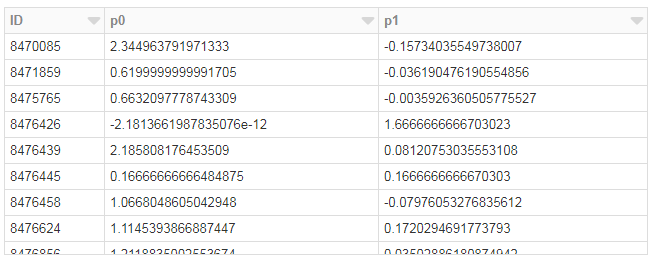### Best Practices

I’ve covered some of the common tasks for using PySpark, but also wanted to provide some advice on making it easier to take the step from Python to PySpark. Here are some of the best practices I’ve collected based on my experience porting a few projects between these environments:

• Avoid dictionaries, use dataframes: using Python data types such as dictionaries means that the code might not be executable in a distributed mode. Instead of using keys to index values in a dictionary, consider adding another column to a dataframe that can be used as a filter.
• Use toPandas sparingly: Calling toPandas() will cause all data to be loaded into memory on the driver node, and prevents operations from being performed in a distributed mode. It’s fine to use this function when data has already been aggregated and you want to make use of familiar Python plotting tools, but it should not be used for large dataframes.
• Avoid for loops: If possible, it’s preferred to rewrite for-loop logic using the groupby-apply pattern to support parallelized code execution. I’ve noticed that focusing on using this pattern in Python has also resulted in cleaning code that is easier to translate to PySpark.
• Try minimizing eager operations: In order for your pipeline to be as scalable as possible, it’s good to avoid eager operations that pull full dataframes into memory. I’ve noticed that reading in CSVs is an eager operation, and my work around is to save the dataframe as parquet and then reload it from parquet to build more scalable pipelines.
• Use framequery/pandasql to make porting easier: If you’re working with someone else’s Python code, it can be tricky to decipher what some of the Pandas operations are achieving. If you plan on porting your code from Python to PySpark, then using a SQL library for Pandas can make this translation easier.

I’ve found that spending time writing code in PySpark has also improved by Python coding skills.

### Conclusion

PySpark is a great language for data scientists to learn because it enables scalable analysis and ML pipelines. If you’re already familiar with Python and Pandas, then much of your knowledge can be applied to Spark. I’ve shown how to perform some common operations with PySpark to bootstrap the learning process. I also showed off some recent Spark functionality with Pandas UDFs that enable Python code to be executed in a distributed mode. There’s great environments that make it easy to get up and running with a Spark cluster, making now a great time to learn PySpark!

Ben Weber is a principal data scientist at Zynga. We are hiring!

February 15, 2019

#### AuthorHakin9 TEAM
Hakin9 is a monthly magazine dedicated to hacking and cybersecurity. In every edition, we try to focus on different approaches to show various techniques - defensive and offensive. This knowledge will help you understand how most popular attacks are performed and how to protect your data from them. Our tutorials, case studies and online courses will prepare you for the upcoming, potential threats in the cyber security world. We collaborate with many individuals and universities and public institutions, but also with companies such as Xento Systems, CATO Networks, EY, CIPHER Intelligence LAB, redBorder, TSG, and others.
Subscribe
Notify ofThis site uses Akismet to reduce spam. Learn how your comment data is processed.

1 Comment
Inline Feedbacks